# Formula Forensics 040 – Apportioning Sales by Criteria

Share

A few weeks back in the Chandoo.org Forum Melvin asked about Apportioning Sales based on Division and Status to the current sales by store.

Today we will examine how this works and how to develop a solution for the problem.

### Apportion/ing

Apportion means to assign or distribute.

In a court the Judge may apportion blame for an accident eg: 50% to the driver, 30% to mechanical failure and 20% to the road conditions, The Judge is assigning or distributing the blame as he deams appropriate.

This is what Melvin wanted to do with his sales. He wanted the sales distributed according to Division and Status based on the current sale by store.

Lets start simple and look at how we can distribute sales on a simple model first.

Let say we have a Distributorship and we buy and sell oranges.

We buy oranges from a supplier and distribute them to 3 stores, Store A, Store B & Store C

We received 1,000 oranges and they were sold as followsWe can see that each store received differing percentages of the original supply: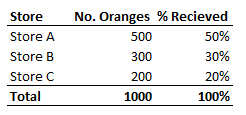50% = 500/1000

30% = 300/1000

etc

A week later the supplier give us another 200 oranges and we want to distribute them based on the previous sales

So the new batch of 200 oranges will be distributed according to these previous percentages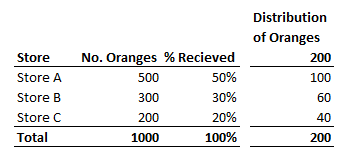100 = 200 x 50%

60 = 200 x 30%

etc

That is as simple and as complicated as apportioning is.

### Melvin’s Problem

When we look at Melvin’s problem he has a more complex set of data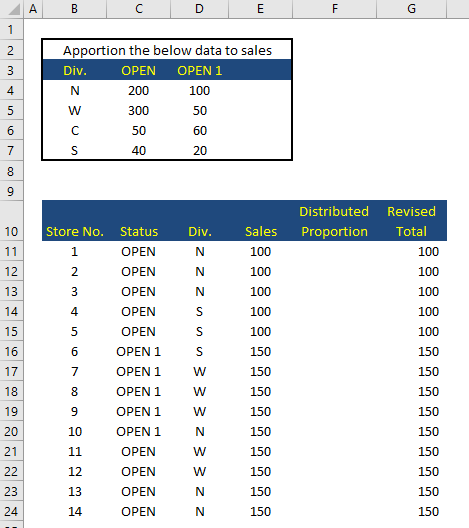We can see that Melvin has 14 stores located in 4 Divisions (N, S, W & C) and each can have a status of Open or Open1

But if we simplify this and look at one set of data we can devise a formula which will adjust to each set of data

Lets develop a formula for cell F11 which is dealing with Store 1 in the N Division and has a Status of Open

We see it has sales of 100 (Cell E11)

Total sales of Division N and status Open are 600 (100+100+100+150+150) highlighted below (Lower table)

Looking at the Upper Table we can see that we need to distribute 200 units based on the Division N and Status Open (Cell C4)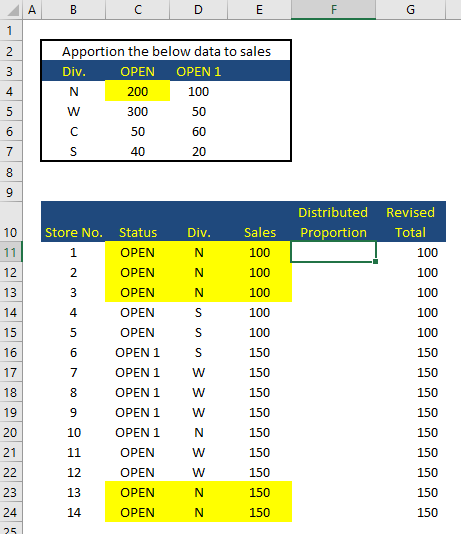So we need to distribute 200 units across the 5 stores with Total sales of 600

We know that Store  1. had sales of 100 in cell E11

The Total Sales of Stores in Division N and Status Open can be obtained using a Sumifs function

=SUMIFS(\$E\$11:\$E\$24, C11:\$C\$24, \$C\$11, \$D\$11:\$D\$24, D11)

=600

So the proportion of Store 1’s sales 100 to Total Sales ( Division N and Status Open ) 600 is 100/600 = 16.66%

This is calculated by

=E11/SUMIFS(\$E\$11:\$E\$24, \$C\$11:\$C\$24, C11, \$D\$11:\$D\$24, D11)

=0.1667

=16.67%

Note: We leave the references to  C11, D11 & E11 variable, so that when the formula is copied down it will refer to the next row

We can use an index/match formula to get the 200 based on the criteria from row 11

=INDEX(\$C\$4:\$D\$7, MATCH(D11,\$B\$4:\$B\$7,0), MATCH(C11,\$C\$3:\$D\$3,0))

What this is doing is doing a 2D Lookup in the Range \$C\$4:\$D\$7

It is looking up the Division Row no. MATCH(D11,\$B\$4:\$B\$7,0)

and looking in the Status Column No. MATCH(C11,\$C\$3:\$D\$3,0)

Note: Once again we leave the references to  C11 & D11 variable, so that when the formula is copied down it will refer to the next row

So the proportion of the 200 sales attributable to Store 1 is:

=Distribution Qty * Actual Sales / Total Sales

=INDEX(\$C\$4:\$D\$7, MATCH(D11, \$B\$4:\$B\$7, 0), MATCH(C11, \$C\$3:\$D\$3, 0)) * E11 / SUMIFS(\$E\$11:\$E\$24, \$C\$11:\$C\$24, C11, \$D\$11:\$D\$24, D11)

= 33.33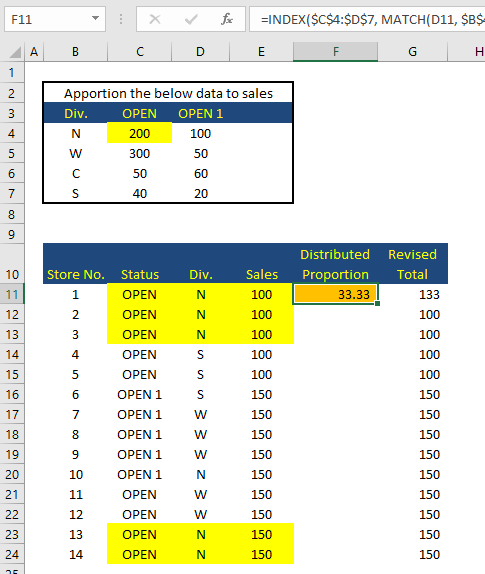We can now copy this down to all the cells matching our criteria of Division N and Status Open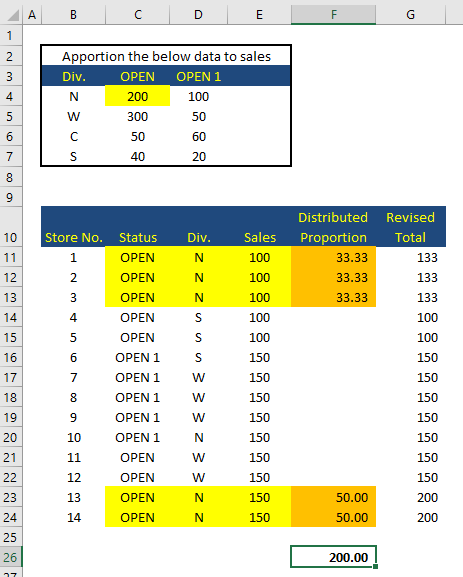Notice that the total matches the total to be distributed 200 showing that the formula is working

Although we copied the formula down to the cells that had matching criteria each part of the formula was setup to work on the appropriate criteria for the store in the current row

If we now copy F11 down to the other stores you will see that in fact all the stores sales have been apportioned according to the correct criteria.eg: If we look at Stores 7, 8 & 9 we can see that they are in the W Division and have a Status of Open1

The distributed Proportions are each 16.67, totaling 50, which matches the distribution in the Upper table.

You may also notice that Division C has not been accounted for.

I assume that Melvin has sent us a subset of the data and that is why it is missing.

## A Challenge

Can you solve the problem another way ?

## Other Posts in this Series

The Formula Forensics Series contains a wealth of useful solutions and information specifically about how Normal Formula and specifically Array Formula work.

If you have a formula and you want to understand how it works contact Hui and it may be featured in future posts.

### Get FREE Excel + Power BI Tips

Simple, fun and useful emails, once per week.

Learn & be awesome.

### Welcome to Chandoo.org

Thank you so much for visiting. My aim is to make you awesome in Excel & Power BI. I do this by sharing videos, tips, examples and downloads on this website. There are more than 1,000 pages with all things Excel, Power BI, Dashboards & VBA here. Go ahead and spend few minutes to be AWESOME.

Read my storyFREE Excel tips book

I will be presenting at two events in London in April 2020. Come & join me.

Chandoo is an awesome teacher
5/5

– Jason

Still on fence about Power BI? In this getting started guide, learn what is Power BI, how to get it and how to create your first report from scratch.

## Related Tips

### 4 Responses to “Formula Forensics 040 – Apportioning Sales by Criteria”

1.MF says:

Here's my attempt:
=VLOOKUP(D11,\$B\$4:\$D\$7,MATCH(C11,\$B\$3:\$D\$3,0),0)*E11/SUMIFS(\$E\$11:\$E\$24,\$C\$11:\$C\$24,C11, \$D\$11:\$D\$24,D11)
Basically the same logic but using VLOOKUP to get the the corresponding number.

2.Mukesh says:

Here My formula

=IF(SUM((\$B\$4:\$B\$7=D11)*(\$C11=C\$3)*(\$C\$4:\$C\$7))/SUM((\$D\$11:\$D\$24=D11)*(\$C\$11:\$C\$24=C11)*(\$E\$11:\$E\$24))*E110,
(SUM((\$B\$4:\$B\$7=D11)*(\$C11=C\$3)*(\$C\$4:\$C\$7))/SUM((\$D\$11:\$D\$24=D11)*(\$C\$11:\$C\$24=C11)*(\$E\$11:\$E\$24))*E11),
(SUM((\$B\$4:\$B\$7=D11)*(\$C11=D\$3)*(\$D\$4:\$D\$7))/SUM((\$D\$11:\$D\$24=D11)*(\$C\$11:\$C\$24=C11)*(\$E\$11:\$E\$24)))*E11)

3.Mukesh says:

Ignore earlier,, Here my correct formula

=IF(SUM((\$B\$4:\$B\$7=D11)*(\$C11=C\$3)*(\$C\$4:\$C\$7))/SUM((\$D\$11:\$D\$24=D11)*(\$C\$11:\$C\$24=C11)*(\$E\$11:\$E\$24))*E110,
(SUM((\$B\$4:\$B\$7=D11)*(\$C11=C\$3)*(\$C\$4:\$C\$7))/SUM((\$D\$11:\$D\$24=D11)*(\$C\$11:\$C\$24=C11)*(\$E\$11:\$E\$24))*E11),
(SUM((\$B\$4:\$B\$7=D11)*(\$C11=D\$3)*(\$D\$4:\$D\$7))/SUM((\$D\$11:\$D\$24=D11)*(\$C\$11:\$C\$24=C11)*(\$E\$11:\$E\$24)))*E11)

4.Walied says:

Well done!

Only for interest, which would you prefer for performance with the same data set but larger data
1. vlookup()
2. match(index())
3. a combination ?

### Get FREE Excel & Power-BI Newsletter

One email per week with Excel and Power BI goodness. Join 100,000+ others and get it free.